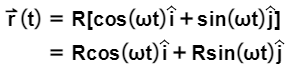###### FREE Expert Solution

Vector magnitude:

$\overline{)\mathbf{|}\stackrel{\mathbf{⇀}}{\mathbit{A}}\mathbf{|}{\mathbf{=}}\sqrt{{{\mathbit{A}}_{\mathbit{x}}}^{\mathbf{2}}\mathbf{+}{{\mathbit{A}}_{\mathbit{y}}}^{\mathbf{2}}}}$

Velocity is:

$\overline{){\mathbf{v}}{\mathbf{=}}\frac{\mathbf{d}\mathbf{r}\mathbf{\left(}\mathbf{t}\mathbf{\right)}}{\mathbf{d}\mathbf{t}}}$

Aceleration:

$\overline{){\mathbf{a}}{\mathbf{=}}\frac{\mathbf{d}\mathbf{v}\mathbf{\left(}\mathbf{t}\mathbf{\right)}}{\mathbf{d}\mathbf{t}}}$

Part A:

The magnitude of r(t) is:

$\begin{array}{rcl}\mathbf{|}\mathbf{r}\mathbf{\left(}\mathbf{t}\mathbf{\right)}\mathbf{|}& \mathbf{=}& \sqrt{{\mathbf{R}}^{\mathbf{2}}\mathbf{c}\mathbf{o}{\mathbf{s}}^{\mathbf{2}}\mathbf{\omega }\mathbf{t}\mathbf{+}{\mathbf{R}}^{\mathbf{2}}\mathbf{s}\mathbf{i}{\mathbf{n}}^{\mathbf{2}}\mathbf{\omega }\mathbf{t}}\end{array}$

81% (32 ratings)###### Problem Details

To find the velocity and acceleration vectors for uniform circular motion and to recognize that this acceleration is the centripedal acceleration.

Suppose that a particle’s position is given by the following expression:Part A. The particle’s motion can be described by ___.
a. an ellipse starting at time t = 0 on the positive x axis
b. an ellipse starting at time t = 0 on the positive y axis
c. a circle starting at time t = 0 on the positive x axis
d. a circle starting at time t = 0 on the positive y axis

Part B. When does the particle first cross the negative x axis?
Express your answer in terms of some or all of the variables ω (Greek letter omega), R, and π.

Part C. Find the particle’s velocity as a function of time.
Express your answer using unit vectors (e.g., A î + B ĵ, where A and B are functions of ω, R, t and π).

Part D. Find the speed of the particle at time t.
Express your answer in terms of some or all of the variables ω, R, and π.

Part E. Now find the acceleration of the particle.
Express your answer using unit vectors (e.g., A î + B ĵ, where A and B are functions of ω, R, t and π).

Part F. Your calculation is actually a derivation of the centripetal acceleration. To see this, express the acceleration of the particle in terms of its position r(t).
Express your answer in terms of some or all of the variables r(t) and ω